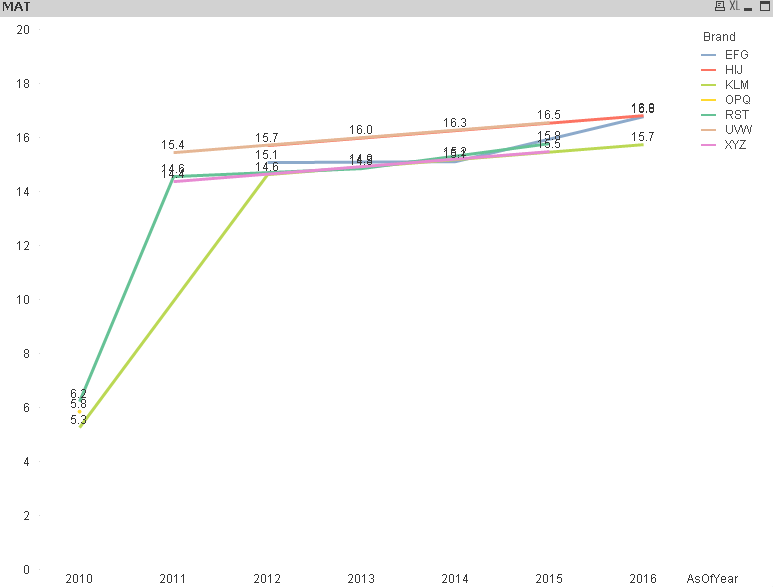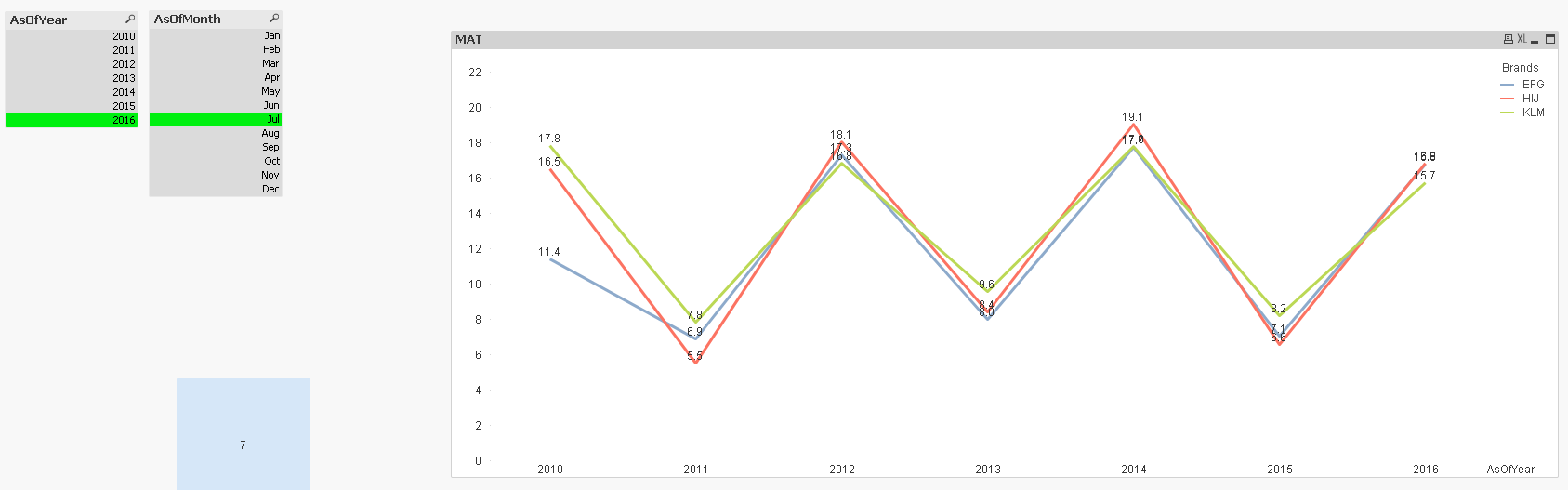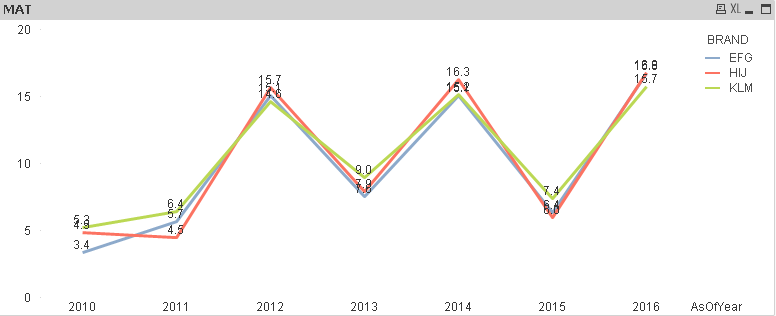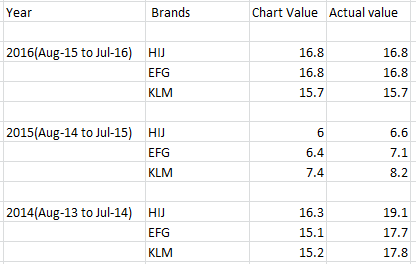# QlikView App Dev

Discussion Board for collaboration related to QlikView App Development.

cancel
Showing results for
Did you mean:Creator III

## To show top 3 Brands

Hi All,

I used below given expression in calculated dimension to show only top 3 brands

=if(aggr(rank(sum({<AsOfYear={'\$(=max(AsOfYear))'}>}Sales)),Brand)<=3,Brand)

and used this expression in expression tab

FirstSortedValue({<AsOfMonthNr = {\$(=Max({<AsOfYear = {\$(=Max(AsOfYear))}>}AsOfMonthNr))},Brand, PeriodType = {'CYMONTH'},  AsOfYear = {"\$(='<=' & Max(AsOfYear))"}, AsOfMonth>}

Aggr(Sum({<Brand, PeriodType = {'CYMONTH'},  AsOfYear, AsOfMonth>}Sales), AsOfPeriod, Brand),

-Aggr(Only({<Brand, PeriodType = {'CYMONTH'},  AsOfYear, AsOfMonth>}AsOfPeriod), AsOfPeriod, Brand))

/

Sum(TOTAL <AsOfPeriod>Aggr(FirstSortedValue({<AsOfMonthNr = {\$(=Max({<AsOfYear = {\$(=Max(AsOfYear))}>}AsOfMonthNr))},Brand, PeriodType = {'CYMONTH'},  AsOfYear = {"\$(='<=' & Max(AsOfYear))"}, AsOfMonth>}

Aggr(Sum({<Brand, PeriodType = {'CYMONTH'}, AsOfYear, AsOfMonth>}Sales), AsOfPeriod, Brand, AsOfYear),

-Aggr(Only({<Brand=, PeriodType = {'CYMONTH'},  AsOfYear, AsOfMonth>}AsOfPeriod), AsOfPeriod, Brand, AsOfYear)), AsOfYear, Brand)))

This expression is work fine the selected year & month  but not for previous for year & month and

This expression works fine when the brand is below 3 or equal to 3 for the selected period.... I mean, only sold brand for the selected period...

There are some other brand also sold in selected period ... The brand share totals 100% when i am trying to show only top 3...

Required output refer the screen shot consider only 2016 valuesAttached the sample data

1 Solution

Accepted SolutionsMVP

Try this

(FirstSortedValue({<AsOfMonthNr = {\$(=Max({<AsOfYear = {\$(=Max(AsOfYear))}>}AsOfMonthNr))},

Brand = {"=Rank(FirstSortedValue({<AsOfMonthNr = {\$(=Max({<AsOfYear = {\$(=Max(AsOfYear))}>}AsOfMonthNr))}, Brand, PeriodType = {'CYMONTH'},  AsOfYear = {'\$(=Max(AsOfYear))'}, AsOfMonth>}

Aggr(Sum({<Brand, PeriodType = {'CYMONTH'},  AsOfYear, AsOfMonth>}Sales), AsOfPeriod, Brand),

-Aggr(Only({<Brand, PeriodType = {'CYMONTH'},  AsOfYear, AsOfMonth>}AsOfPeriod), AsOfPeriod, Brand))) <= 3"},

PeriodType = {'CYMONTH'},  AsOfYear = {"\$(='<=' & Max(AsOfYear))"}, AsOfMonth>}

Aggr(Sum({<Brand, PeriodType = {'CYMONTH'},  AsOfYear, AsOfMonth>}Sales), AsOfPeriod, Brand),

-Aggr(Only({<Brand, PeriodType = {'CYMONTH'},  AsOfYear, AsOfMonth>}AsOfPeriod), AsOfPeriod, Brand))

/

Sum({<AsOfMonthNr = {\$(=Max({<AsOfYear = {\$(=Max(AsOfYear))}>}AsOfMonthNr))},Brand , PeriodType = {'CYMONTH'},  AsOfYear = {"\$(='<=' & Max(AsOfYear))"}, AsOfMonth>}TOTAL <AsOfYear>Aggr(FirstSortedValue({<AsOfMonthNr = {\$(=Max({<AsOfYear = {\$(=Max(AsOfYear))}>}AsOfMonthNr))},Brand , PeriodType = {'CYMONTH'},  AsOfYear = {"\$(='<=' & Max(AsOfYear))"}, AsOfMonth>}

Aggr(Sum({<Brand, PeriodType = {'CYMONTH'}, AsOfYear, AsOfMonth>}Sales), AsOfPeriod, Brand, AsOfYear),

-Aggr(Only({<Brand=, PeriodType = {'CYMONTH'},  AsOfYear, AsOfMonth>}AsOfPeriod), AsOfPeriod, Brand, AsOfYear)), AsOfYear, Brand)))*1008 RepliesCreator III
Author

Hi All

I made a correction in calculated dimension my  selected year & month show correct values but my previous year of same month not  show correct values

=if(aggr(rank(

sum({<AsOfPeriod ={"\$(='>=' & Date(AddMonths(Max(AsOfPeriod), -11), 'MMM-YY') & '<=' & Date(Max(AsOfPeriod), 'MMM-YY'))"},PeriodType={'CYMONTH'}>}Sales)),Brand)<=3,Brand)

Can any one help me......MVP

Seems you have done aggr before rank only. May be this?

If(Aggr(Rank(sum({<AsOfPeriod ={">=\$(=Date(AddMonths(Max(AsOfPeriod), -11), 'MMM-YY')) <= \$(Date(Max(AsOfPeriod), 'MMM-YY'))"},PeriodType={'CYMONTH'}>}Sales))<=3, Brand), Brand)

Before develop something, think If placed (The Right information | To the right people | At the Right time | In the Right place | With the Right context)Creator III
Author

No Brother ... its not working.... same values are showing

Get outputCreator III
Author

Required Ouput values areCreator III
Author

Anil...

can help what is the error in this expression

Brand = {"=rank(sum({<AsOfPeriod ={"\$(='>=' & Date(AddMonths(Max(AsOfPeriod), -11), 'MMM-YY') & '<=' & Date(Max(AsOfPeriod), 'MMM-YY'))"}>}Sales)) <= 3"}

when i tired to insert it with main one it has some error.....MVP

Try this

(FirstSortedValue({<AsOfMonthNr = {\$(=Max({<AsOfYear = {\$(=Max(AsOfYear))}>}AsOfMonthNr))},

Brand = {"=Rank(FirstSortedValue({<AsOfMonthNr = {\$(=Max({<AsOfYear = {\$(=Max(AsOfYear))}>}AsOfMonthNr))}, Brand, PeriodType = {'CYMONTH'},  AsOfYear = {'\$(=Max(AsOfYear))'}, AsOfMonth>}

Aggr(Sum({<Brand, PeriodType = {'CYMONTH'},  AsOfYear, AsOfMonth>}Sales), AsOfPeriod, Brand),

-Aggr(Only({<Brand, PeriodType = {'CYMONTH'},  AsOfYear, AsOfMonth>}AsOfPeriod), AsOfPeriod, Brand))) <= 3"},

PeriodType = {'CYMONTH'},  AsOfYear = {"\$(='<=' & Max(AsOfYear))"}, AsOfMonth>}

Aggr(Sum({<Brand, PeriodType = {'CYMONTH'},  AsOfYear, AsOfMonth>}Sales), AsOfPeriod, Brand),

-Aggr(Only({<Brand, PeriodType = {'CYMONTH'},  AsOfYear, AsOfMonth>}AsOfPeriod), AsOfPeriod, Brand))

/

Sum({<AsOfMonthNr = {\$(=Max({<AsOfYear = {\$(=Max(AsOfYear))}>}AsOfMonthNr))},Brand , PeriodType = {'CYMONTH'},  AsOfYear = {"\$(='<=' & Max(AsOfYear))"}, AsOfMonth>}TOTAL <AsOfYear>Aggr(FirstSortedValue({<AsOfMonthNr = {\$(=Max({<AsOfYear = {\$(=Max(AsOfYear))}>}AsOfMonthNr))},Brand , PeriodType = {'CYMONTH'},  AsOfYear = {"\$(='<=' & Max(AsOfYear))"}, AsOfMonth>}

Aggr(Sum({<Brand, PeriodType = {'CYMONTH'}, AsOfYear, AsOfMonth>}Sales), AsOfPeriod, Brand, AsOfYear),

-Aggr(Only({<Brand=, PeriodType = {'CYMONTH'},  AsOfYear, AsOfMonth>}AsOfPeriod), AsOfPeriod, Brand, AsOfYear)), AsOfYear, Brand)))*100Creator III
Author

yeah brother.... its working fine ....thank you very much.........MVP

No problem